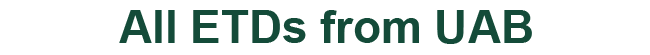### Resonant Solutions to Gross-Pitaevskii Equation With Periodic Potential

Yulia Karpeshina

Ryoichi Kawai

Ian Knowles

Boris Kunin

Kabe Moen

Dissertation

2022

#### Degree Name by School

Doctor of Philosophy (PhD) College of Arts and Sciences

#### Abstract

Over the years, many methods have been used for solving the Gross-Pitaevskii equation which is also known as a nonlinear Schrodinger equation. In this thesis, we study a nonlinear polyharmonic equation with a periodic potential and quasi-periodic boundary conditions in dimensions higher than one. A special case of the equation, in two dimensions, is the Gross-Pitaevskii equation. This thesis aims to find solutions close to combinations of two plane waves at high energy. To start with, we use a technique similar to that known for the linear case. Then, we construct a method of successive approximations. We obtain the desired solutions as the limit of the successive approximation procedure.

COinS